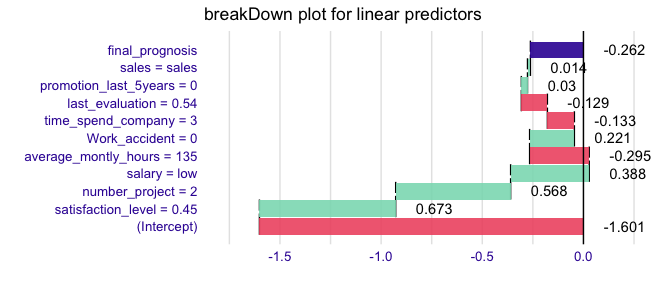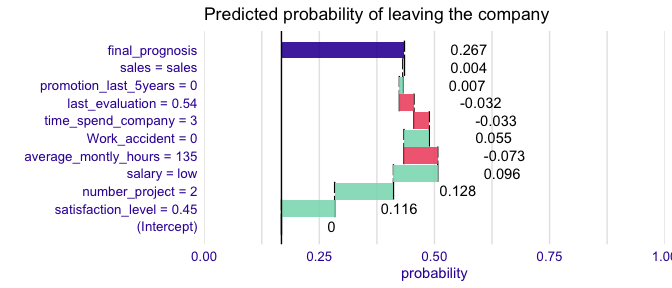# breakDown plots for the generalised linear models

#### 2020-04-04

Here we will use the HR churn data (https://www.kaggle.com/) to present the breakDown package for glm models.

The data is in the breakDown package

library(breakDown)
#>   satisfaction_level last_evaluation number_project average_montly_hours
#> 1               0.38            0.53              2                  157
#> 2               0.80            0.86              5                  262
#> 3               0.11            0.88              7                  272
#>   time_spend_company Work_accident left promotion_last_5years sales salary
#> 1                  3             0    1                     0 sales    low
#> 2                  6             0    1                     0 sales medium
#> 3                  4             0    1                     0 sales medium

Now let’s create a logistic regression model for churn, the left variable.

model <- glm(left~., data = HR_data, family = "binomial")

But how to understand which factors drive predictions for a single observation?

With the breakDown package!

Explanations for the linear predictor.

library(ggplot2)
#>        11
#> -0.262138

explain_1 <- broken(model, HR_data[11,])
explain_1
#>                            contribution
#> (Intercept)                      -1.601
#> satisfaction_level = 0.45         0.673
#> number_project = 2                0.568
#> salary = low                      0.388
#> average_montly_hours = 135       -0.295
#> Work_accident = 0                 0.221
#> time_spend_company = 3           -0.133
#> last_evaluation = 0.54           -0.129
#> promotion_last_5years = 0         0.030
#> sales = sales                     0.014
#> final_prognosis                  -0.262
#> baseline:  0
plot(explain_1) + ggtitle("breakDown plot for linear predictors")Explanations for the probability with intercept set as an origin.

predict(model, HR_data[11,], type = "response")
#>        11
#> 0.4348382

explain_1 <- broken(model, HR_data[11,], baseline = "intercept")
explain_1
#>                            contribution
#> (Intercept)                       0.000
#> satisfaction_level = 0.45         0.673
#> number_project = 2                0.568
#> salary = low                      0.388
#> average_montly_hours = 135       -0.295
#> Work_accident = 0                 0.221
#> time_spend_company = 3           -0.133
#> last_evaluation = 0.54           -0.129
#> promotion_last_5years = 0         0.030
#> sales = sales                     0.014
#> final_prognosis                   1.339
#> baseline:  -1.601457
plot(explain_1,
trans = function(x) exp(x)/(1+exp(x))) + ggtitle("Predicted probability of leaving the company")+ scale_y_continuous( limits = c(0,1), name = "probability", expand = c(0,0))
#> Scale for 'y' is already present. Adding another scale for 'y', which will
#> replace the existing scale.